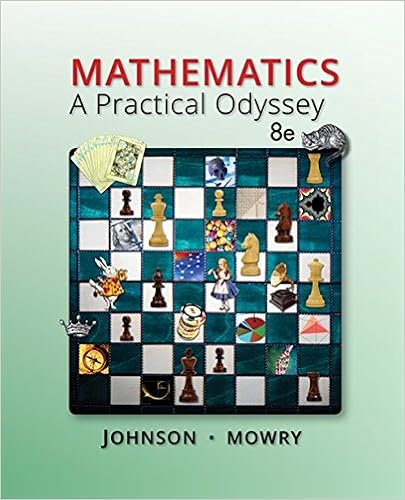# Test1_Review.pdf - Test 1 Review MA 114-003 Spring 2017...

• Notes
• 4

This preview shows page 1 - 2 out of 4 pages.

##### We have textbook solutions for you!
The document you are viewing contains questions related to this textbook.The document you are viewing contains questions related to this textbook.
Chapter 11 / Exercise 45
Mathematics: A Practical Odyssey
Johnson/MowryExpert Verified
Test 1 ReviewMA 114-003Spring 2017Test 1 will cover sections 1.1-1.4, 2.1-2.5, and 3.1-3.2 inFinite Mathematicsand its Applications, Goldstein, Schneider, and Siegel, 11th Edition.TopicsEquations of lines: slope-intercept/standard form, and point-slope formGraphing lines: using 2 ”easy” points or slope and y-interceptGraphing linear inequalitiesFinding feasible set of a system of linear inequalitiesFinding the intersection point of a pair of linesGauss-Jordan elimination method with pivotingSolving systems of linear equations with more than 2 variables using an aug-mented matrix and Gauss-Jordan elimination with pivotsIdentifying whether the system has a unique solution, infinitely many solutions,or no solutionMatrix arithmeticAdding and subtracting matrices: matrix sizes need to agreeScalar multiplicationMultiplying matrices: inner dimensions need to agreeMatrix inverseFinding inverse of 2×2 matrix: using determinant and formulaFinding inverse of matrix of larger dimension: using Gauss-Jordan elimination
##### We have textbook solutions for you!
The document you are viewing contains questions related to this textbook.The document you are viewing contains questions related to this textbook.
Chapter 11 / Exercise 45
Mathematics: A Practical Odyssey
Johnson/MowryExpert Verified
•••